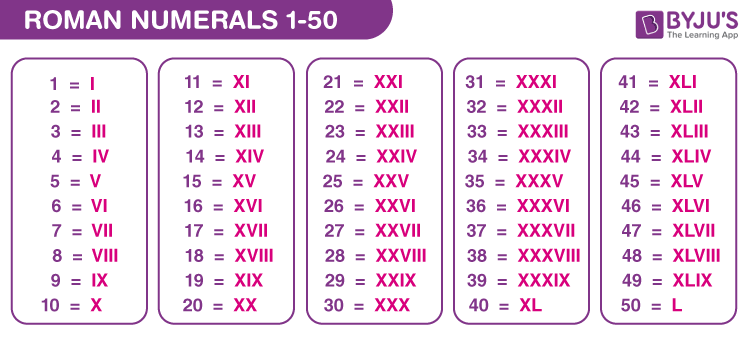Roman numerals from 1 to 50 are I, II, III, IV, V,…, X,…, L. The list of roman numerals from 1 to 50 contains the roman letters, along with equivalent numbers from 1 to 50. This list of roman numerals is used to learn the natural numbers from 1 to 50, with their respective roman letter representations. In this article, you will learn the roman numerals from 1 to 50, and you can also download the chart for roman numerals 1 to 50 in a PDF format.

Understand more about Roman numerals here.

## Roman Numerals 1 to 50 Chart

Below is the chart for roman numerals 1 to 50.Also, try: Roman numerals Converter

## List of Roman Numerals 1 to 50

The below table shows the equivalent roman numerals for the numbers from 1 to 50.

 1 to 25 in Roman Numerals 26 to 50 in Roman Numerals Number Expansion Roman Numeral Number Roman Numeral 1 1 I 26 10 + 10 + 5 + 1 XXVI 2 1 + 1 II 27 10 + 10 + 5 + 1 + 1 XXVII 3 1 + 1 + 1 III 28 10 + 10 + 5 + 1 + 1 + 1 XXVIII 4 5 – 1 IV 29 10 + 10 – 1 + 10 XXIX 5 5 V 30 10 + 10 + 10 XXX 6 5 + 1 VI 31 10 + 10 + 10 + 1 XXXI 7 5 + 1 + 1 VII 32 10 + 10 + 10 + 1 + 1 XXXII 8 5 + 1 + 1 + 1 VIII 33 10 + 10 + 10 + 1 + 1 + 1 XXXIII 9 10 – 1 IX 34 10 + 10 + 10 – 1 + 5 XXXIV 10 10 X 35 10 + 10 + 10 + 5 XXXV 11 10 + 1 XI 36 10 + 10 + 10 + 5 + 1 XXXVI 12 10 + 1 + 1 XII 37 10 + 10 + 10 + 5 + 1 + 1 XXXVII 13 10 + 1 + 1 + 1 XIII 38 10 + 10 + 10 + 5 + 1 + 1 + 1 XXXVIII 14 10 – 1 + 5 XIV 39 10 + 10 + 10 – 1 + 10 XXXIX 15 10 + 5 XV 40 50 – 10 XL 16 10 + 5 + 1 XVI 41 50 – 10 + 1 XLI 17 10 + 5 + 1 + 1 XVII 42 50 – 10 + 1 + 1 XLII 18 10 + 5 + 1 + 1 + 1 XVIII 43 50 – 10 + 1 + 1 + 1 XLIII 19 10 – 1 + 10 XIX 44 50 – 10 – 1 + 5 XLIV 20 10 + 10 XX 45 50 – 10 + 5 XLV 21 10 + 10 + 1 XXI 46 50 – 10 + 5 + 1 XLVI 22 10 + 10 + 1 + 1 XXII 47 50 – 10 + 5 + 1 + 1 XLVII 23 10 + 10 + 1 + 1 + 1 XXIII 48 50 – 10 + 5 + 1 + 1 + 1 XLVIII 24 10 + 10 – 1 + 5 XXIV 49 50 – 10 – 1 + 10 XLIX 25 10 + 10 + 5 XXV 50 50 L

## Video Lesson on Roman Numerals## How to Write Roman Numerals for Numbers 1 to 50?

The sequence of roman numerals is given below:

I, II, III, IV, V, VI, VII, VIII, IX, X, XI, XII, XIII, XIV, XV, XVI, XVII, XVIII, XIX, XX, XXI, XXII,…

From the above, we can derive the following basic rules for the conversion of roman numerals into numerical values.

• When a bigger letter precedes a smaller letter, we should subtract the corresponding numerical values. For example, the value of XL can be derived as L – X = 50 – 10 = 40, where L is the bigger number.
• Also, the notation XL can be read as “ten less than fifty, i.e. 40”.
• When a bigger letter succeeds a smaller letter, we should add the corresponding numerical values. Consider LX, where X is the bigger number. So, LX = L + X = 50 + 10 = 60. Also, the notation LX can be read as “ten more than fifty, i.e. 60”.

## Frequently Asked Questions on Roman Numerals 1 to 50

### How do you write 50 in roman numerals?

In roman numerals, the number 50 can be represented using a specific letter. 50 in roman numeral representation is L, i.e., L = 50.

### Write 40 in roman numerals.

40 in roman numerals can be written using subtraction of letters. That means, 40 = 50 – 10, i.e. 40 = L – X = XL. Therefore, 40 in roman numerals is XL.

### What number is XVII in roman numerals?

XVII = X + V + I + I = 10 + 5 + 1 + 1 = 17
The number 17 is equivalent to XVII in roman numerals.

### What does XXXIV mean in roman numerals?

XXXIV = X + X + X – 1 + 5 = 10 + 10 + 10 – 1 + 5 = 34
Hence, the number 34 is equivalent to XXXIV in roman numerals.